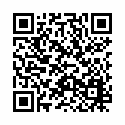## Math Formula Solution SimulatorMath Formula Solution Simulator

Math Formula Simulator - You don't like to solve a mathematical formula? Or just can't find the right solution?
Consider increasing the formula IQ!
Raffle friends, show your scanner mathematical formulas, decide even complex formula!
The control is very easy to move the camera to the formula and press the scan, any difficult task you will be easy!
Attention to draw the game and fun designed!
The application cannot give the right answer to a mathematical formula!
Thank you for playing with us, let us know your feedback and review, and we will make our game even better!

User Reviews
Based on 0 Votes and 0 User Reviews
5 Star
0
4 Star
0
3 Star
0
2 Star
0
1 Star
0